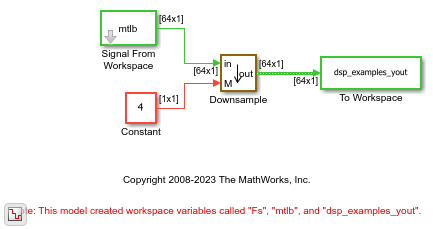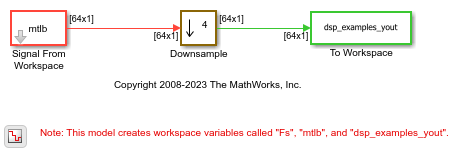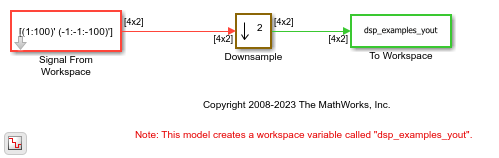# Downsample

Resample input at lower rate by deleting samples

•Libraries:
DSP System Toolbox / Signal Operations
DSP System Toolbox HDL Support / Signal Operations

## Description

The Downsample block decreases the sampling rate of the input by deleting samples. When the block performs frame-based processing, it resamples the data in each column of the Pi-by-Q input matrix independently. When the block performs sample-based processing, it treats each element of the input as a separate channel and resamples each channel of the input array across time. The resample rate is M times lower than the input sample rate, where M is the value of the downsample factor. The Downsample block resamples the input by discarding M–1 consecutive samples following each sample that is output.

This block supports triggered subsystems when you set the Rate options parameter to ```Enforce single-rate processing```.

## Examples

expand all

In this example, the Downsample block decreases the sample rate by decreasing the frame size.

Open the model. The Downsample block resamples a single-channel input with a frame size of 64. In the dialog box of the Downsample block, the Downsample factor source parameter is set to `Input port`. This sets the Input processing and the Rate options parameters automatically to `Columns as Channels (frame based)` and `Enforce single-rate processing`, respectively. Specify a downsample factor of 4 from the input port.

Run the model. The block operates in the single-rate processing mode and downsamples the input by a factor of 4. The output of the block has a frame size of 64. As the block operates in the single-rate processing mode, the input and output signals of the Downsample block have the same frame rate. You can confirm this from the Timing Legend. To open the legend, click the Debug tab on the model toolstrip and click Information Overlays > Timing Legend.In this example, the Downsample block decreases the sample rate by decreasing the frame rate.

Open the model. The input to the Downsample block is a single-channel signal with a frame period of one second. In the block dialog box, set the Downsample factor, M to 4 and Rate options to `Allow multirate processing`.

Run the model. The block operates in the multirate frame-based processing mode. With a downsample factor of 4, the output of the block has a frame period of 4 seconds. You can confirm this from the Timing Legend. To open the legend, click the Debug tab on the model toolstrip and click Information Overlays > Timing Legend. As the block operates in the multirate processing mode, the input and output signals of the Downsample block have the same frame size.Downsample a signal by a factor of 2 using the Downsample block.

Open the System and Run the ModelThe Signal From Workspace block generates a two-channel signal with a frame size of 4. The timing legend shows the sample time of the signal before and after the downsampling operation. To see the legend, click the Debug tab > Information Overlapys > Timing Legend.

Run the model. The sample time of the signal before the downsampling operation is half the sample time of the signal after the operation.

One-Frame Latency

The Input processing parameter in the Downsample block is set to `Columns as channels (frame based)` and the input frame size (number of rows in the input) Mi is greater than 1. Hence, the latency of the signal is one frame. The Initial conditions parameter is set to `[11 -11;12 -12;13 -13;14 -14]`. In all cases of one-frame latency, the Mi rows of the initial condition matrix appear in sequence as the first four output rows. Input sample D+1 (that is, row D+1 of the input matrix) appears in the output as sample Mi+1, followed by input sample D+1+K, input sample D+1+2K, and so on.

• Mi - Number of input rows. In this example, Mi equals 4.

• D - Sample offset parameter. In this example, D equals 1.

• K - Downsample factor. In this example, K equals 2.

The Initial conditions value can be an Mi-by- N matrix containing one value for each channel, or a scalar to be repeated across all elements of the Mi-by- N matrix.

Here is the downsampled output signal written to the `dsp_examples_yout` variable in the base workspace.

```dsp_examples_yout = 11 -11 12 -12 13 -13 14 -14 2 -2 4 -4 6 -6 8 -8 10 -10 12 -12 14 -14 16 -16 18 -18 20 -20 22 -22 24 -24 26 -26 28 -28 30 -30 32 -32 34 -34 36 -36 38 -38 40 -40 42 -42 44 -44 46 -46 48 -48 50 -50 52 -52 54 -54 56 -56 58 -58 60 -60 62 -62 64 -64 66 -66 68 -68 70 -70 72 -72 74 -74 76 -76 78 -78 80 -80 82 -82 84 -84 86 -86 88 -88 90 -90 92 -92 94 -94 96 -96 98 -98 100 -100 0 0 0 0 0 0 0 0 0 0 0 0 0 0 0 0 0 0 0 0 ```

## Ports

### Input

expand all

Input data for which you want to decrease the sample rate, specified as a column vector or a matrix.

When you set the Input processing parameter to `Elements as channels (sample based)`, the input can be an N-D array.

The block supports variable-size input signals when you set Input processing to ```Columns as channels (frame based)``` and Rate options to ```Enforce single-rate processing```, so the frame length (number of rows) and the number of channels (columns) can change during simulation. When the block accepts variable-size input signals, they can be of arbitrary frame length, that is, the input frame length does not have to be a multiple of the downsampling factor. When you specify fixed-size signals, the frame length can be arbitrary under certain conditions. For more details, see Frame-Based Processing and Sample-Based Processing.

This port is unnamed until you set Downsample factor source to ```Input port```. (since R2023a)

Data Types: `single` | `double` | `int8` | `int16` | `int32` | `int64` | `uint8` | `uint16` | `uint32` | `uint64` | `Boolean` | `fixed point`
Complex Number Support: Yes

Since R2023a

Specify the downsample factor M as a positive integer.

#### Dependency

To enable this port, set the Downsample factor source parameter to ```Input port```.

Data Types: `single` | `double` | `int8` | `int16` | `int32` | `uint8` | `uint16` | `uint32`

### Output

expand all

Downsampled output with a sampling rate that is 1/M times the input sampling rate, returned as a column vector or a matrix.

When you set Rate options to:

• `Enforce single-rate processing` –– The block maintains the input sample rate and downsamples the signal by decreasing the output frame size by a factor of M.

The output has an upper bound size of `ceil`(P/M)-by-Q for an input of size P-by-Q.

• `Allow multirate processing` –– The block downsamples the signal such that the output sample rate is M times slower than the input sample rate.

The output frame size is the same as the input frame size.

For more details, see Frame-Based Processing and Sample-Based Processing.

The output is a variable-size signal when any of these conditions are met:

• The input is a variable-size signal.

• The input is a fixed-size signal and the Allow arbitrary frame length for fixed-size input signals parameter is selected.

• Downsample factor is input through the port M (since R2023a)

When the output is a variable-size signal, the block maintains the phase across consecutive frames of data. For more details, see Phase Continuity.

Data Types: `single` | `double` | `int8` | `int16` | `int32` | `int64` | `uint8` | `uint16` | `uint32` | `uint64` | `Boolean` | `fixed point`
Complex Number Support: Yes

## Parameters

expand all

Since R2023a

Specify the downsample factor through one of these options:

• `Dialog parameter` –– The block enables the Downsample factor, M parameter in the block dialog box.

• `Input port` –– The block enables the input port M through which you specify the downsample factor.

Specify the integer factor M by which to decrease the input sample rate.

#### Dependency

To enable this parameter, set Downsample factor source to ```Dialog parameter```. (since R2023a)

The Sample offset (0 to M−1) parameter delays the output samples by an integer number of sample periods, D, where 0 ≤ D ≤ (M–1), so that you can select any of the M possible output phases. For example, when you downsample the sequence 1, 2, 3, ... by a factor of 4, you can select from four phases.

Input SequenceSample Offset, DOutput Sequence (M = 4)

`1,2,3,...`

0

`1,5,9,13,17,21,25,29,...`

`1,2,3,...`

1

`0,2,6,10,14,18,22,26,...`

`1,2,3,...`

2

`0,3,7,11,15,19,23,27,...`

`1,2,3,...`

3

`0,4,8,12,16,20,24,28,...`

The initial zero in the last three output sequences in the table is a result of the Initial conditions parameter being set to its default value of 0. See Latency for more information on the Initial conditions parameter.

When you input the downsample factor through the input port M, the Sample offset (0 to M−1) parameter is not enabled in the block dialog box. In this case, the sample offset D is automatically set to 0. (since R2023a)

#### Dependency

To enable this parameter, set Downsample factor source to ```Dialog parameter```. (since R2023a)

Specify the method for input processing:

• `Columns as channels (frame based)` –– When you set the Input processing parameter to `Columns as channels (frame based)`, the block treats each of the Q input columns as an individual channel containing Pi sequential time samples. The block downsamples each channel independently by discarding M–1 rows of the input matrix following each row that it outputs.

• `Elements as channels (sample based)` –– When you set the Input processing parameter to `Elements as channels (sample based)`, the input can be a Q-D array. The Downsample block treats each element of the input as a separate channel, and resamples each channel of the input over time. The block downsamples the input array by discarding M–1 samples following each sample that it passes through to the output. The input and output sizes of the Downsample block are identical.

#### Dependency

To enable this parameter, set Downsample factor source to ```Dialog parameter```. (since R2023a)

When you set Downsample factor source to ```Input port```, the Input processing parameter is automatically set to ```Columns as channels (frame based)```. (since R2023a)

Specify the method by which the block adjusts the rate at the output port to accommodate the reduced number of samples. The block performs single-rate or multirate processing depending on whether you set the Input processing parameter to ```Elements as channels (sample based)``` (sample-based processing mode) or `Columns as channels (frame based)` (frame-based processing mode).

• ```Elements as channels (sample based)```

• ```Enforce single-rate processing```

The block forces the output sample rate to match the input sample rate (Tso = Tsi) by repeating every Mth input sample M times at the output. In this mode, the block behaves like the Sample and Hold block with a repeating trigger event of period MTsi.

• ```Allow multirate processing```

The sample period of the output is M times longer than the input sample period (Tso = MTsi).

For more details, see Sample-Based Processing

• ```Columns as channels (frame based)```

• ```Enforce single rate processing```

The block generates the output at the slower (downsampled) rate using a proportionally smaller frame size than the input. The output has an upper bound size of `ceil`(P/M), where P is the input frame length and M is the downsample factor. The input and output frame rates are equal. For more details, see Frame-Based Processing. For an example, see Downsample Signal in Single-Rate Frame-Based Processing Mode.

• ```Allow multirate processing```

The block generates the output at the slower (downsampled) rate by using a proportionally longer frame period at the output port than at the input port. For downsampling by a factor of M, the output frame period is M times longer than the input frame period (Tfo = MTfi), but the input and output frame sizes are equal. For more details, see Frame-Based Processing. For an example, see Downsample Signal In Multirate Frame-Based Processing Mode.

#### Dependency

To enable this parameter, set Downsample factor source to ```Dialog parameter```. (since R2023a)

When you set Downsample factor source to ```Input port```, the Rate options parameter is automatically set to ```Enforce single-rate processing```. (since R2023a)

Specify whether fixed-size input signals (size does not change during simulation) can have an arbitrary frame length, where the frame length does not have to be a multiple of the downsampling factor. The block uses this parameter setting only for fixed-size input signals and ignores this parameter if the input has a variable size.

When the input signal is a variable-size signal, the signal can have an arbitrary frame length, that is, the frame length does not have to be a multiple of the decimation factor.

For fixed-size input signals, if you:

• Select the Allow arbitrary frame length for fixed-size input signals parameter, the frame length of the signal does not have to be a multiple of the downsampling factor. If the input is not a multiple of the downsampling factor, then the output is generally a variable-size signal. Therefore, to support an arbitrary input size, the block must also support variable-size operations, which you can enable by selecting the Allow arbitrary frame length for fixed-size input signals parameter.

• Clear the Allow arbitrary frame length for fixed-size input signals parameter, the input frame length must be a multiple of the downsampling factor.

#### Dependency

To enable this parameter, set:

• Downsample factor source to ```Dialog parameter```. (since R2023a)

• Input processing to ```Columns as channels (frame based)```.

• Rate options to ```Enforce single-rate processing```.

The initial block value for cases of nonzero latency. You can specify a scalar or an array of the same size as the input.

#### Dependencies

This parameter does not appear when Input processing is set to ```Columns as channels (frame based)``` and Rate options is set to `Enforce single-rate processing`.

## Block Characteristics

 Data Types `Boolean` | `double` | `fixed point` | `integer` | `single` Direct Feedthrough `no` Multidimensional Signals `no` Variable-Size Signals `yes` Zero-Crossing Detection `no`

expand all

## Version History

Introduced before R2006a

expand all